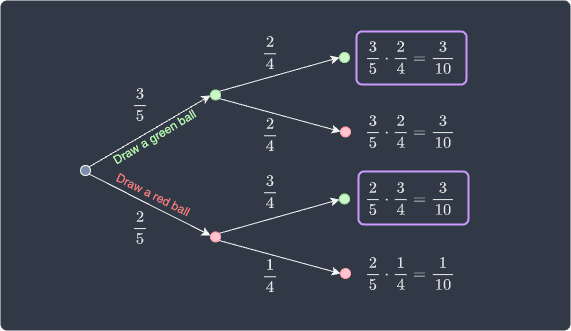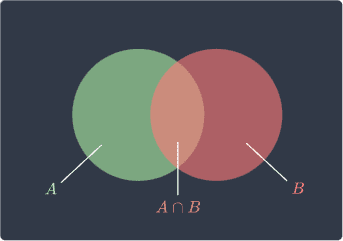search
Search
Unlock 100+ guides
search toc
close
account_circle
Profile
exit_to_app
Sign out
search
keyboard_voice
close
Searching Tips
Search for a recipe:
"Creating a table in MySQL"
Search for an API documentation: "@append"
Search for code: "!dataframe"
Apply a tag filter: "#python"
Useful Shortcuts
/ to open search panel
Esc to close search panel
to navigate between search results
d to clear all current filters
Enter to expand content previewDoc SearchCode Search BetaSORRY NOTHING FOUND!
mic
Start speaking...Voice search is only supported in Safari and Chrome.
Shrink
Navigate to

# Multiplication Rule and Addition Rule in Probability Theory

schedule Aug 11, 2023
Last updated
local_offer
Probability and Statistics
Tags
mode_heat
Master the mathematics behind data science with 100+ top-tier guides
Start your free 7-days trial now!
Theorem.

# Multiplication rule

If $A$ and $B$ are events, then the probability of both $A$ and $B$ occurring is:

\begin{align*} \mathbb{P}(A,B) &=\mathbb{P}(A)\cdot{\mathbb{P}(B\vert{A})}\\ &=\mathbb{P}(B)\cdot{\mathbb{P}(A\vert{B})} \end{align*}

Proof. The multiplication rule is simply a rearranged version of the formula of conditional probabilitylink:

\begin{align*} \mathbb{P}(B|A)=\frac{\mathbb{P}(A,B)}{\mathbb{P}(A)} \;\;\;\;\;\Longleftrightarrow\;\;\;\;\; \mathbb{P}(A,B)=\mathbb{P}(B|A)\cdot{\mathbb{P}(A)}\\ \end{align*}

This completes the proof.

Example.

## Drawing a green ball from a bag

Suppose we have a bag containing $5$ balls of which $3$ are green and $2$ are red. Suppose we draw two balls in succession from this bag without replacement. Answer the following:

• what is the probability of drawing $2$ green balls?

• what is the probability of drawing a red ball followed by a green ball?

Solution. Let's define the following events:

• event $\color{green}G_1$ - selecting a green ball for the first draw.

• event $\color{green}G_2$ - selecting a green ball for the second draw.

• event $\color{red}R_1$ - selecting a red ball for the first draw.

The probability of drawing $2$ green balls is:

\begin{align*} \mathbb{P}({\color{green}G_1},{\color{green}G_2})&= \mathbb{P}({\color{green}G_1}) \cdot{\mathbb{P}({\color{green}G_2}\vert{\color{green}G_1})}\\ &=\frac{3}{5}\cdot\frac{2}{4}\\ &=\frac{3}{10} \end{align*}

The probability of drawing a red ball followed by a green ball is:

\begin{align*} \mathbb{P}({\color{red}R_1},{\color{green}G_2})&= \mathbb{P}({\color{red}R_1}) \cdot{\mathbb{P}({\color{green}G_2}\vert{\color{red}R_1})}\\ &=\frac{2}{5}\cdot\frac{3}{4}\\ &=\frac{3}{10} \end{align*}

We've directly applied the formula for the multiplication rule of probability here, but we don't have to blindly apply the formula. We can approach this problem using a probability tree diagram as well:Here, the top bounded box is the probability of drawing two green balls and the bottom bounded box is the probability of drawing a red ball first followed by a green ball.

Theorem.

# Multiplication rule of independent events

If $A$ and $B$ are independent events, then:

$$\mathbb{P}(A,B)= \mathbb{P}(A)\cdot\mathbb{P}(B)$$

Proof. The multiplication rule for probabilities is:

$$\begin{equation}\label{eq:KNmyJfG7wjdt6LKwHg2} \mathbb{P}(A,B)=\mathbb{P}(A|B)\cdot\mathbb{P}(B) \end{equation}$$

If events $A$ and $B$ are independent, then this means that the probability of $A$ is not affected by the occurrence of $B$, which means that $\mathbb{P}(A\vert{B}) = \mathbb{P}(A)$. Therefore \eqref{eq:KNmyJfG7wjdt6LKwHg2} becomes:

$$\mathbb{P}(A,B)=\mathbb{P}(A)\cdot\mathbb{P}(B)$$

This completes the proof.

Example.

## Rolling two fair dices

Suppose we roll two fair dices. What is the probability that the outcome of both the dices is even?

Solution. Let $A$ and $B$ represent the outcome of the first and second dice respectively. The events $(A\text{ is even})$ and $(B\text{ is even})$ are independent because the outcome of the first dice does not affect the outcome of the second dice. Therefore, the probability that the outcomes of both dices are even is:

\begin{align*} \mathbb{P}(A\text{ is even },B\text{ is even}) &=\mathbb{P}(A\text{ is even})\cdot\mathbb{P}(B\text{ is even})\\ &=\frac{3}{6}\cdot\frac{3}{6}\\ &=\frac{1}{4} \end{align*}
Theorem.

# Addition rule in probability theory

If $A$ and $B$ are two events, then the probability that either one of them will occur is:

$$\mathbb{P}(A\text{ or }{B})=\mathbb{P}(A)+\mathbb{P}(B)-\mathbb{P}(A\text{ and }B)$$

In set notation, this is expressed as:

$$\mathbb{P}(A\cup{B}) =\mathbb{P}(A)+\mathbb{P}(B)-\mathbb{P}(A\cap{B})$$

Where $\cup$ represents union and $\cap$ represents intersection.

Proof. We can prove the addition rule by referring to the Venn diagram below:Let's start by confirming that the following is true:

$$A\cup{B}=(A\setminus{B})\cup{B}$$

Here, the $\setminus$ means exclusion. Taking the probability of both sides gives:

$$\mathbb{P}(A\cup{B}) = \mathbb{P}\Big((A\setminus{B})\cup{B}\Big)$$

Since $A\setminus{B}$ and $B$ are disjoint, the third axiom of probabilitylink tells us that:

$$\mathbb{P}(A\cup{B}) = \mathbb{P}(A\setminus{B})+\mathbb{P}(B)$$

But $A\setminus{B}=A\setminus(A\cap{B})$ so that:

$$\begin{equation}\label{eq:msEow9fqvJTNGWPgHqj} \mathbb{P}(A\cup{B}) = \mathbb{P}\Big(A\setminus{(A\cap{B})}\Big)+\mathbb{P}(B) \end{equation}$$

Now, refer to the Venn diagram again:Confirm that the following is true:

$$A= \Big(A\setminus(A\cap{B})\Big)\cup(A\cap{B})$$

Taking the probability of both sides gives:

$$\mathbb{P}(A)= \mathbb{P}\Big[\Big(A\setminus(A\cap{B})\Big)\cup(A\cap{B})\Big]$$

Again, $A\setminus(A\cap{B})$ and $A\cap{B}$ are disjoint, and so by the third axiom of probability, we have that:

$$\mathbb{P}(A)= \mathbb{P}\Big(A\setminus(A\cap{B})\Big) +\mathbb{P}(A\cap{B})$$

We rearrange this like so:

$$\begin{equation}\label{eq:PbZPnEWakXHqQISG5kU} \mathbb{P}\Big(A\setminus(A\cap{B})\Big) = \mathbb{P}(A)-\mathbb{P}(A\cap{B}) \end{equation}$$

Finally, substituting \eqref{eq:PbZPnEWakXHqQISG5kU} into \eqref{eq:msEow9fqvJTNGWPgHqj} gives us the addition rule:

$$\mathbb{P}(A\cup{B}) = \mathbb{P}(A)+\mathbb{P}(B)-\mathbb{P}(A\cap{B})$$

This completes the proof.

Example.

## Rolling a dice once

Suppose we roll a fair dice once. What is the probability that the outcome is even or prime?

Solution. We define the following two events:

• event $A$ - outcome is even $\{2,4,6\}$.

• event $B$ - outcome is prime $\{2,3,5\}$.

Notice how rolling a $2$ satisfies both events, that is, $A\cap{B}=\{2\}$. Now, let's apply the addition rule:

\begin{align*} \mathbb{P}(A\text{ or }{B})&=\mathbb{P}(A)+\mathbb{P}(B)-\mathbb{P}(A\text{ and }B)\\ &=\frac{3}{6}+\frac{3}{6}-\frac{1}{6}\\ &=\frac{5}{6} \end{align*}

Here, we must subtract by $\mathbb{P}(A\text{ and }B)$ because we are double counting the probability of getting a $2$.

Example.

## Drawing a card from a deck of cards

Suppose we randomly draw a single card from a standard deck of $52$ cards. Find the probability that the card is a hearts or red.

Solution. We define the following two events:

• event $A$ - the drawn card is a hearts.

• event $B$ - the drawn card is red.

A standard deck contains $13$ hearts and $26$ red cards, of which half are hearts. Therefore, we are double counting all the hearts, which means that there are $13$ cards in the set $A\cap{B}$, that is, $\vert{A\cap{B}}\vert=13$. Let's apply the addition rule:

\begin{align*} \mathbb{P}(A\text{ or }{B})&=\mathbb{P}(A)+\mathbb{P}(B)-\mathbb{P}(A\text{ and }B)\\ &=\frac{13}{52}+\frac{26}{52}-\frac{13}{52}\\ &=\frac{1}{2} \end{align*}
Theorem.

# Addition rule when events are mutually exclusive

If $A$ and $B$ are two mutually exclusive events, then:

$$\mathbb{P}(A\text{ or }{B})=\mathbb{P}(A)+ \mathbb{P}(B)$$

Proof. We know from the addition rule of probabilities that:

$$\begin{equation}\label{eq:UOGUODp6B3g5DYYudoV} \mathbb{P}(A\text{ or }{B})=\mathbb{P}(A)+\mathbb{P}(B)-\mathbb{P}(A\text{ and }B) \end{equation}$$

If $A$ and $B$ are two mutually exclusive events, then $\mathbb{P}(A\text{ and }B)=0$ because $A$ and $B$ can never occur together. Therefore, \eqref{eq:UOGUODp6B3g5DYYudoV} reduces down to:

$$\mathbb{P}(A\text{ or }{B})=\mathbb{P}(A)+\mathbb{P}(B)$$

This completes the proof.

Example.

## Rolling a dice once (mutually exclusive events)

Suppose we roll a fair dice once. Find the probability of rolling a $1$ or $2$.

Solution. Let's define the following events:

• event $A$ - rolling a $1$.

• event $B$ - rolling a $2$.

Clearly, these events are mutually exclusive because they cannot happen simultaneously, that is, we can never roll both a $1$ and a $2$ with a single roll. We now apply the addition rule for mutually exclusive events:

\begin{align*} \mathbb{P}(A\text{ or }{B})&=\mathbb{P}(A)+\mathbb{P}(B)\\ &=\frac{1}{6}+\frac{1}{6}\\ &=\frac{1}{3} \end{align*}
thumb_up
thumb_down
Comment
Citation
Ask a question or leave a feedback...
thumb_up
0
thumb_down
0
chat_bubble_outline
0
settings
Enjoy our search
Hit / to insta-search docs and recipes!